StatLect

# Joint distribution function

The joint distribution function is a function that completely characterizes the probability distribution of a random vector.## Synonyms and acronyms

It is also called joint cumulative distribution function (abbreviated as joint cdf).

## Joint cdf of X and Y

Let us start with the simple case in which we have two random variablesand.

Their joint cdf is defined aswhereandare two real numbers.

Note that:

•indicates a probability;

• the comma inside the parentheses stands for AND.

In other words, the joint cdfgives the probability that two conditions are simultaneously true:

• the random variabletakes a value less than or equal to;

• the random variabletakes a value less than or equal to.

## Example

Suppose that there are only four possible cases:Further assume that each of these cases has probability equal to 1/4.

Let us compute, as an example, the following value of the joint distribution function:The two conditions that need to be simultaneously true are:There are two cases in which they are satisfied:Therefore, we have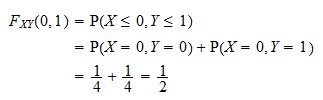## The formula for discrete variables

In the previous example we have shown a special case.

In general, the formula for the joint cdf of two discrete random variablesandis: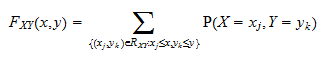where:

•is the support of the vector, that is, the set of all the values ofthat have a strictly positive probability of being observed;

• we sum the probabilities over the setthat contains all the couplesbelonging to the support and such thatand.

The probabilities in the sum are often written using the so-called joint probability mass function## How to compute the formula with a table

The sum in the formula above can be easily computed with the help of a table.

Here is an example.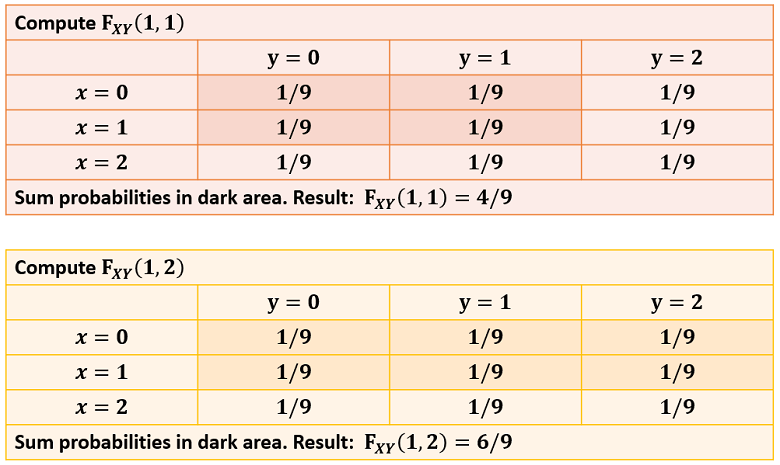In this table, there are nine possible couplesand they all have the same probability (1/9).

In order to compute the joint cumulative distribution function, all we need to do is to shade all the probabilities to the left of(included) and above(included).

Then, the value ofis equal to the sum of the probabilities in the shaded area.

## The formula for continuous variables

Whenandare continuous random variables, we need to use the formula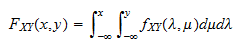whereis the joint probability density function ofand.

The computation of the double integral can be broken down in two steps:

1. first compute the inner integralwhich, in general, is a function ofand;

2. then calculate the outer integral### Example

Let us make an example.

Let the joint pdf be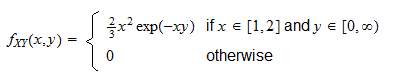Whenand, we have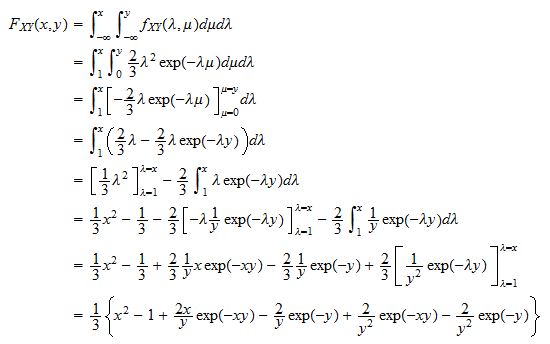This is only one of the possible cases. We also have the two cases:

1.or, in which case2.and, in which case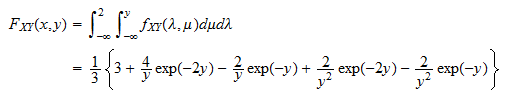## How to derive the marginal cdfs from the joint

The two marginal distribution functions ofandareThey can be derived from the joint cumulative distribution function as follows:where the exact meaning of the notation isThis can be demonstrated as follows: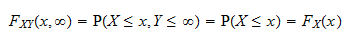because the conditionis always met and, as a consequence, the conditionis satisfied wheneveris true.

The proof foris analogous.

## Deriving the joint cdf from the marginals

In general, we cannot derive the joint cdf from the marginals, unless we know the so-called copula function, which links the two marginals.

However, there is an important exception, discussed in the next section.

## Joint cdf of two independent variables

Whenandare independent, then the joint cdf is equal to the product of the marginals:See the lecture on independent random variables for a proof, a discussion and some examples.

## A more general definition

Until now, we have discussed the case of two random variables. However, the joint cdf is defined for any collection of random variables forming a random vector.

Definition The joint distribution function of arandom vectoris a functionsuch that: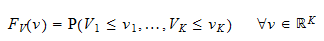where the entries ofandare denoted byandrespectively, for.

## More details

More details about joint distribution functions can be found in the lecture entitled Random vectors.

Previous entry: Integrable random variable

Next entry: Joint probability density function